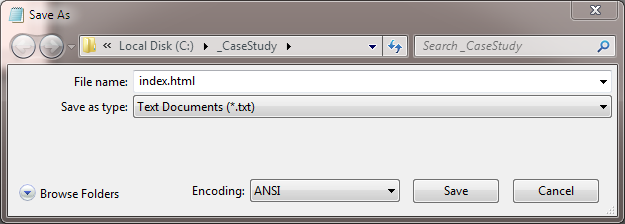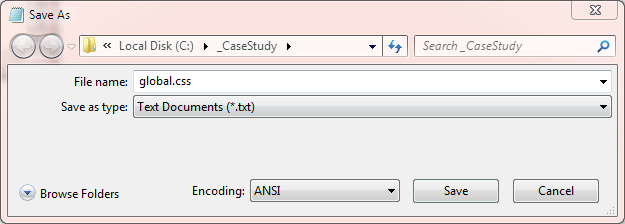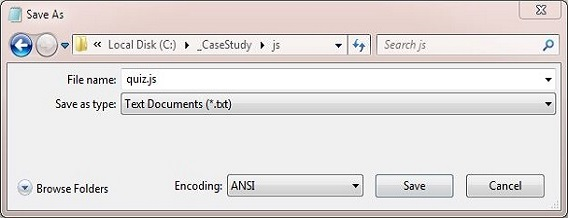## Enhancing The Quiz

In this lesson we enhance the interactive quiz to dynamically calculate the number of questions and give better feedback on quiz completion.

We now have a working quiz but when the results are shown they just say 'right' or 'wrong' and don't give the user an indication of the correct answer when they get a question wrong. We can alter the code so we give a correct answer for any questions the user gets wrong. This will improve user feedback and help with the learning.

Another thing we can improve is the progress bar and how we calculate a users progress through the quiz. Currently this is hardcoded so if we change the number of questions then we have to change the calculations for the progress bar as we navigate forwards and backwards though the quiz. Although we are not going to change the correct answers array which is hardcoded at the moment, at a future point we might want to import the correct answers from a file. By dynamically calculating the number of questions on page load we could then make this enhancement at a later date and just remove the hardcoded array.

So we have several tasks to complete to achieve these aims:

2. When navigating forwards through the quiz calculate the number of questions from our hardcoded answers array to calculate the width of the progress bar.
3. When navigating backwards through the quiz extract the question number from the question container to calculate the width of the progress bar.
4. On quiz completion:
1. Extract correct answers for any wrong question to result set.
2. Remove hardcoded number of questions from result set and calculate this instead.

### Updating Our HTML

We need to add explanations for each answer in case the user gets a question wrong, so we can show this in the results container.

Using Notepad reopen the `index.html` file we updated in Lesson 2: Quiz Layout.

Copy and paste the following code into the reopened file, between the opening </body> and closing </body> element overwriting the existing HTML.

``````
<div id="main">
<h1>Interactive Quiz</h1>
<div class="questionContainer" style="display: block;">
<div class="question"><strong>Question 1 :</strong> What goes inside a class definition?</div>
<ul><li><label><input type="radio" id="q1-a" name="q1" /> Functions</label></li>
<li><label><input type="radio" id="q1-b" name="q1" /> Subroutines</label></li>
<li><label><input type="radio" id="q1-c" name="q1" /> Methods</label></li>
<li><label><input type="radio" id="q1-d" name="q1" /> Procedures</label></li></ul>
</div>
<div class="hide red" id="exp1"> - Methods go inside a class definition.</div>
<div class="btnContainer">
<div class="prev"></div>
<div class="next"><a class="btnNext">Next ++</a></div>
<div class="clear"></div>
</div>
</div>
<div class="questionContainer hide">
<div class="question"><strong>Question 2 :</strong> java command compiles?</div>
<ul><li><label><input type="radio" id="q2-a" name="q2" /> true</label></li>
<li><label><input type="radio" id="q2-b" name="q2" /> false</label></li></ul>
</div>
<div class="hide red" id="exp2"> - We use the javac command to compile programs.</div>
<div class="btnContainer">
<div class="prev"><a class="btnPrev">-- Prev</a></div>
<div class="next"><a class="btnNext">Next ++</a></div>
<div class="clear"></div>
</div>
</div>
<div class="questionContainer hide">
<div class="question"><strong>Question 3 :</strong> A statement contains methods?</div>
<ul><li><label><input type="radio" id="q3-a" name="q3" /> true</label></li>
<li><label><input type="radio" id="q3-b" name="q3" /> false</label></li></ul>
</div>
<div class="hide red" id="exp3"> - A method contains statements.</div>
<div class="btnContainer">
<div class="prev"><a class="btnPrev">-- Prev</a></div>
<div class="next"><a class="btnShowResult">Finish</a></div>
<div class="clear"></div>
</div>
</div>
<div class="txtStatusBar">Quiz Progress Bar
<div id="progressContainer" style="display: block;">
<div id="progress"></div>
</div>
<div class="hide" id="resultContainer"></div>
</div>
``````

Save the file in the C:\_CaseStudy folder and close the Notepad.### Reviewing The HTML

We have added divisions for our question explanations within each question container.

### Updating Our Layout

Using Notepad or a simlilar text editor, reopen the `global.css` we created in Lesson 2: Quiz Layout.

We only need to add the CSS for question explanations and we will also highlight the score, so copy and paste the following CSS to the end of the existing file..

``````

/* Question explanation and score highlight */
.red {color:red;}
.totalScore{font-weight:bold;}

``````

Save the file in the C:\_CaseStudy folder and close the Notepad.### Updating Quiz JavaScript File

We can now make the updates to our javaScript file.

Using Notepad make sure you are pointing to the `C:\_CaseStudy\js` folder and reopen the `quiz.js` file we created in Lesson 4: Quiz Navigation.

Copy and paste the following code into the reopened file overwriting the contents. The JavaScript and jQuery we have added for this lessons enhancements are shown in green:

``````
// JavaScript and jQuery for interactive quiz follows
\$(function(){

var S2CQuiz = {
init: function(){
\$('.btnNext').on('click', function(){
return false;
}
});
var progBar = \$('#progress');
var numq = S2CQuiz.numQuestions();
var progWidth = 720 / numq;
progBar.width(progBar.width() + progWidth + 'px');
});
\$('.btnPrev').on('click', function(){
});
var progBar = \$('#progress');
// Extract question number and calculate width
var qString =  \$(this).parents('.questionContainer').children()
.first().children('strong').text();
var numq = parseInt(qString.substr(9, 2));
numq--;
var progWidth = progBar.width() / numq;
progBar.width(progBar.width() - progWidth + 'px');
});
\$('.btnShowResult').on('click', function(){
return false;
}
\$('#progress').width(0);
\$('#progressContainer').hide();
\$('.txtStatusBar').text('Quiz Result');
var ans = S2CQuiz.userAnswers = [];
for (var i = 0; i < arr.length; i++) {
ans.push(arr[i].getAttribute('id'));
}
var resultSet = '';
var rightCount = 0;
for (var i = 0; i < results.length; i++){
if (results[i] == "right") {
rightCount++;
resultSet += '<div> Question ' + (i + 1) + ' is ' + results[i] + '</div>';
} else {
var expid = '#' + 'exp' + (i + 1);
var exp = \$(expid).text();
resultSet += '<div class="red"> Question ' + (i + 1) + ' is ' + results[i]
+ '<strong>' + exp + '</strong></div>';
}
}
var numq = S2CQuiz.numQuestions();
resultSet += '<div class="totalScore">You scored ' + rightCount + '/' +  numq + '</div>';
\$('#resultContainer').html(resultSet).show();
});
},
answers: { q1: 'c', q2: 'b', q3: 'b' },
// Check answers and output 'right' or 'wrong' to another array
var resultArr = [];
for (var p in ans) {
var x = parseInt(p) + 1;
var key = 'q' + x;
var flag = "wrong";
if (ans[p] == 'q' + x + '-' + arr[key]) {
flag = "right";
}
else {
flag = "wrong";
}
resultArr.push(flag);
}
return resultArr;
},
// Calcualte number of question
numQuestions: function() {
var x = 0;
for (var p in arr) {
if (p < 10) {
x = parseInt(p.substr(1, 1));
} else {
x = parseInt(p.substr(1, 2));
}
}
return x;
}
};
S2CQuiz.init();
});
``````

Save the file in the C:\_CaseStudy\js folder and close the Notepad.### Reviewing The JavaScript and jQuery

When the user navigates forward through the quiz we calculate the number of questions and just divide this by the size of the progress bar. We then use the `.width()` properties method to update the progress bar. When the user navigates backwards through the quiz we use various jQuery methods to extract `Question xx` from the `.question` division and extract the number. We then use the number with the `.width()` properties method to update the progress bar.

For the results feedback when an answer is wrong we extract the explanation using the `.text()` inner DOM insertion method and output this to the result set.

### Viewing Our Changes

From the C:\_CaseStudy folder, double click on the `index.html` saved file and the quiz will appear in your default web browser. Navigate through the quiz answering the questions and making sure you answer some incorrectly. Press the `.btnShowResult` link and you will see a screenshot similar to the following showing the results of the quiz:.## Lesson 6 Complete

In this lesson we refined our layout.